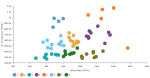# DCPY.SPECTRALCLUST(n_clusters, random_state, n_init, columns)

Spectral clustering works by applying K-means clustering on fewer dimensions as a result of low-dimension embedding of the affinity matrix between data points.

###### Parameters
• n_clusters – Number of clusters to find, integer (default 8).

• random_state – Seed used to generate random numbers by the K-means initialization and eigenvectors decomposition, integer (default 0).

• n_init – Number of times the K-means will run with different centroids seeds, to get the best output, integer (default 10).

• columns – Dataset columns or custom calculations.

Example: DCPY.SPECTRALCLUST(8, 0, 10, sum([Gross Sales]), sum([No of customers])) used as a calculation for the Color field of the Scatterplot visualization.###### Input data
• Numeric variables are automatically scaled to zero mean and unit variance.
• Character variables are transformed to numeric values using one-hot encoding.
• Dates are treated as character variables, so they are also one-hot encoded.
• Size of input data is not limited, but many categories in character or date variables increase rapidly the dimensionality.
• Rows that contain missing values in any of their columns are dropped.
###### Result
• Column of integer values starting with 0, where each number corresponds to a cluster assigned to each record (row) by the algorithm.
• Rows that were dropped from input data due to containing missing values have missing value instead of assigned cluster.
###### Key usage points
• It often outperforms traditional clustering methods like K-means.
• Very useful when the structure of individual clusters is highly non-convex or when a measure of the center and spread of the cluster is not a suitable description of the complete cluster, for example nested circles on the 2D plan.
• Works well when the estimated number of clusters is relatively low.
• Avoid using it with too many clusters.
• Must know estimated number of clusters.
• Lower clustering quality when the dataset contains structures at different scales of size and density.
• High time complexity and memory usage, suitable for small to medium sized datasets.
###### Example

For the whole list of algorithms, see Data science built-in algorithms.